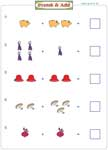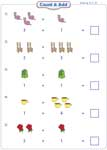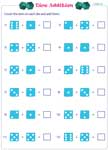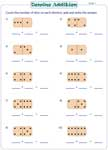Picture addition worksheets provide intensive practice on counting and adding pictures; addition with dice and domino; word problems with pictures and more. Coloring activities and teacher templates are also included. For Kindergarten through 2nd grade.

Count and add the Pictures

Count the pictures and add them to find their sum in each problem. The worksheets have colorful pictures to make the activity more interesting for kids.Sums up to 5:

Sums up to 10:

Sums up to 20:

Complete the addition equation

Complete the addition equation by either counting the pictures or adding the cardinalities given for each group of pictures.Sums up to 5:

Sums up to 10:

Sums up to 20:

Write the addition sentence

Write the addition sentence by counting the pictures in each group. Children can practice picture addition with sums up to 20 in these worksheets.Sums up to 5:

Sums up to 10:

Sums up to 20:

Follow the directions and color the shapes accordingly. Count the colored shapes and add them together to find their sum. Also, write the addition sentence.Sums up to 5:

Sums up to 10:

Sums up to 20:

Repeated addition word problems

Kids can practice repeated addition in these worksheets. Each worksheet has five basic word problems with attractive pictures.Count the dots on each die and add to find their sum. The first three sheets have two dice and the next three sheets have three dice in each problem.Two dice:

Three dice:

Domino is one of the great way to practice addition. Kids should find the sum in each problem by counting and adding the dots on each domino.Teacher Templates

Home-school moms, tutors and teachers may use these templates to make their own worksheets based on dice and dominoes.Dice:

Domino:

Domino Template

The whole batch of worksheets on addition with pictures can be downloaded instantly.

Related Worksheets